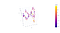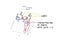Topological Data Analysis

Simplicical Complexes, the origin of everyhting.

The effect of connecting points in a space as increasing some radius around them results in the creation of geometric objects called simplices. Intuitively, a simplicial complex structure on a space is an expression of the space as a union of points, intervals, triangles and other higher dimensional analogues (1). This action of connecting points is called filtration. Filtration will control how data points are transformed into simplicial complexes in order to transform sets of points into a topological space. The building blocks of a simplicial complex are called simplices (plural of simplex).

Persistence Homology basics

Homology refers to a class of methods using simplicical complexes to study topologies. It allows us to infer topological information from intrinsic geometric properties of the objects. A we already pointed in our introduction, what we do is to replace the role of data objects by its network of relationships. The decomposition of a space into path-connected components is a qualitative property of this space. As we can see in above figure, when increasing radius some connections between points appears. First connections draws lines as goes from pont to point. With higher radius values we can get new expressions as for example loops. Let’s use an example to explain what is homology.

Betti numbers and persistence entropy

Betti numbers (denoted by β) are a formalization of the count of the number of independent k-dimensional surfaces in space. For example, β0 refers to the number of connected components (zeroth-level connectivity information) , β1 is the number of one dimensional or “circular” holes (level-one connectivity information) and β2 is the number of two-dimensional “voids” or “cavities”. Betti numbers are a vector or signature of integers (β0, β1, β2) descibring the space. This vector is called data’s topologica signature. In figure 3, object “4” signatures are β0=1, β1=1 (1, 1) and in object “8” , β0=1, β1=2 (1, 2). Betti number varies with the value of the radius (or filtration parameter or scale parameter -denoted by ε-).

Mapper algorithm

Mapper algorithm is a topological multi-step method to visualize geometric objects (4). Visualization means qualitative representation, so mapper is not a data analysis algorithm itself. It can be used to generate a topological graph that captures the salient features of the data. The advantages of mapper are it’s numbness to metrics, understanding sensitivity to parameter changes and its multiscale approach.

Hands on with COVID-19 drug discovery data set

The Government of India made this dataset publicly accessible as part of their Drug Discovery Hackathon. It includes a list of drugs that have been tested and their effectiveness against Sars-CoV-2. D. Agrawal completed this list by adding some molecule chemical details obtained from PubChem. The final dataset includes 100 checked molecules, each with 40 features. Among features included in dataset we have pIC50, the negative logarithm of the half maximal inhibitory concentration (IC50). This index is used as a measure of the efficacy of a substance in inhibiting a specific biological or biochemical function. Our goal is to find a classification method for this molecules according it’s effectiveness against Sars-CoV-2. The dataset also contains five blinded molecules, which do not show the pIC50 value.

Python libraries

We will use the Giotto-tda library to perform computations. gtda is a high-performance topological machine learning toolbox written in Python.
The real advantage of using gtda, in my opinion, is its direct integration with scikit-learn and support for tabular data, time series, graphs, or pictures.
Both assets make it simple to construct machine learning pipelines.

STEP 1. Building a point cloud

We have a high dimensional space (100 x 40) that can be considered as a point cloud. To visualize this dataset, we have two options: visualize only the first two or three features (for a 2d or 3d plot) and compute the high dimensional data withmapper , or perform a dimensionality reduction to two or three components. Let’s begin with strategy number two.

features = clean.loc[:, :'pIC50']
n_neighbors=10
min_dist=0.5
umap_2d = umap.UMAP(n_neighbors=n_neighbors
n_components=2,
min_dist=min_dist,
init='random',
random_state=0)
umap_3d = umap.UMAP(n_neighbors=n_neighbors,
n_components=3,
min_dist=min_dist,
init='random',
random_state=0)
proj_2d = umap_2d.fit_transform(clean.drop(columns='pIC50'))
proj_3d = umap_3d.fit_transform(clean.drop(columns='pIC50'))
fig_2d = px.scatter( proj_2d, x=0, y=1,
color=clean['pIC50'],
labels={'color': 'pIC50'}
)
fig_3d = px.scatter_3d(proj_3d, x=0, y=1, z=2,
color=clean['pIC50'],
labels={'color': 'pIC50'}
)
fig_2d.update_layout(title='UMAP projection 2D and 3D')
fig_3d.update_traces(marker_size=5)
fig_2d.update_layout({'plot_bgcolor': 'aliceblue' , 'paper_bgcolor': 'white',}, template='plotly_white')fig_3d.update_layout({'plot_bgcolor': 'aliceblue' , 'paper_bgcolor': 'white',}, template='plotly_white')fig_2d.show()
fig_3d.show()Figure 8. Dataset weighted graph with UMAP. Color scale corresponds to pIC50 valuesFigure 9. Dataset weighted graph with UMAP. Color scale corresponds to binarized pIC50 values

STEP 2. Visualization with Mapper algorithm

1. define a covering,
2. and compute clustering.

Filter function

gtda offers three alternatives: projection , entropy and eccentrycity . My suggestion for a point cloud is to choose eccentrycity as offers better filtration resolution. Default distance funtion metric for eccentrycity is euclidean distance. We have chosen this default metric.

Covering

We have two options: CubicalCoverning for multi-dimensional data and OneDimensionCover for one-dimension data. CubicalCoverning has to important choices: number of intervales and overlap fractions.

Cluster

The default cluster algorithm is scikit-learn DBSCAN. The most important choice we have to make is epsilon parameter eps, it is, the maximum distance between two samples for one to be considered as in the neighborhood of the other. Results from mapper algorithm depends entirely from this parameter. Due to its importance, we are going to use some metrics to check the separation distance between the resulting DBSCAN clusters. In our example we are going to use Silhouette analysis.

Mapper

Mapper algorith builds a pipeline with parameters described above:

#build a pipeline for mapper algorithm
make_mapper_pipeline(filter_func,
cover,
clusterer)
""" 1. Define filter function – can be any scikit-learn transformer.It is returning a selection of columns of the data """filter_func = Eccentricity(metric= 'euclidean') #Eccentricities of points in a point cloud or abstract metric space.""" 2. Define cover """
cover = CubicalCover(n_intervals=30, overlap_frac=0.3)
""" 3. Choose clustering algorithm – default is DBSCAN """
clusterer = DBSCAN(eps=8, min_samples=3, metric='euclidean')
""" 4. Initialise pipeline """
pipe_mapper = make_mapper_pipeline(
filter_func=filter_func, cover=cover, clusterer=clusterer, verbose=False, n_jobs=-1
)
data = clean.drop(columns='pIC50')#Check the cluster performance
db = clusterer.fit(data)
labels = db.labels_
n_clusters_ = len(set(labels)) - (1 if -1 in labels else 0)
n_noise_ = list(labels).count(-1)
print('Estimated number of clusters: %d' % n_clusters_)
print('Estimated number of noise points: %d' % n_noise_)
"""The best value of Silhouette score is 1, and the worst value is -1. Values near 0 indicate overlapping clusters. Negative values generally indicate that a sample has been assigned to the wrong cluster, as a different cluster is more similar."""print("Silhouette Coefficient: %0.3f" % metrics.silhouette_score(proj_3d, labels))
Estimated number of clusters: 1
Estimated number of noise points: 88
Silhouette Coefficient: 0.003
plotly_params = {"node_trace": {"marker_colorscale": "RdBu"}}fig = plot_static_mapper_graph(
pipe_mapper, data, layout='fruchterman_reingold', color_by_columns_dropdown=True, color_variable =clean['pIC50'], node_scale =20, plotly_params=plotly_params
)
fig.show(config={'scrollZoom': True})